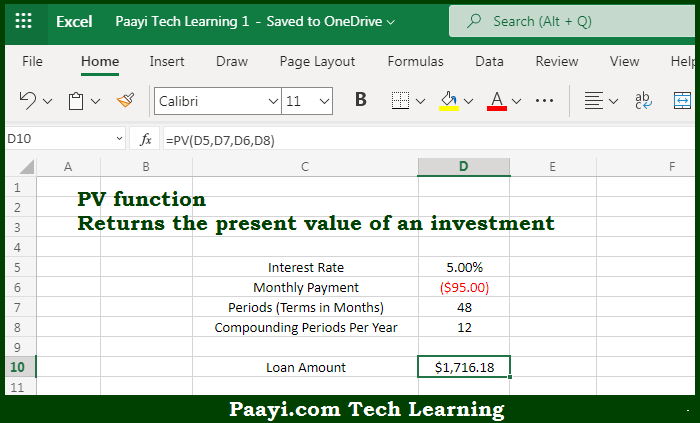# Learn How to Use Microsoft Excel PV Function

Written by | 0 Comments | 523 Views

In this article, you will learn how to use the Microsoft Excel PV function and its prime function in Microsoft Excel. You will also get to know the Microsoft Excel PV function return value and syntax with the help of some examples.

Microsoft Excel PV Function

The main purpose of the Microsoft Excel PV function is to get the present value of the investment. That implies, with the help of the PV function you can able to return the present value of an investment. The PV function can be used to get the value in today's dollars of a series of future payments, assuming periodic, constant payments and a constant interest rate. So, with the help of the PV function, you can able to get the present value of an investment.

Return Value of PV Function

The return value will be the present value.

Syntax of PV Function

=PV(rate, nper, pmt, [fv], [type])

Where the arguments:

• rate: This is the interest rate per period.
• nper: This is the total number of payment periods.
• pmt: This is the payment made each period.
• fv: This is the cash balance you want to get after the last payment is made. In case not, it is assumed to be zero.
• type: This is used when payments are due, where 0 = end of the period, and 1 = beginning of the period. The default is 0.

How to Use Microsoft Excel PV Function?So we know that Microsoft Excel PV function you can able to get the present value of the investment. That implies, with the help of the PV function you can able to return the present value of an investment. The PV function can be used to get the value in today's dollars of a series of future payments, assuming periodic, constant payments and a constant interest rate. So, with the help of the PV function, you can able to get the present value of an investment.